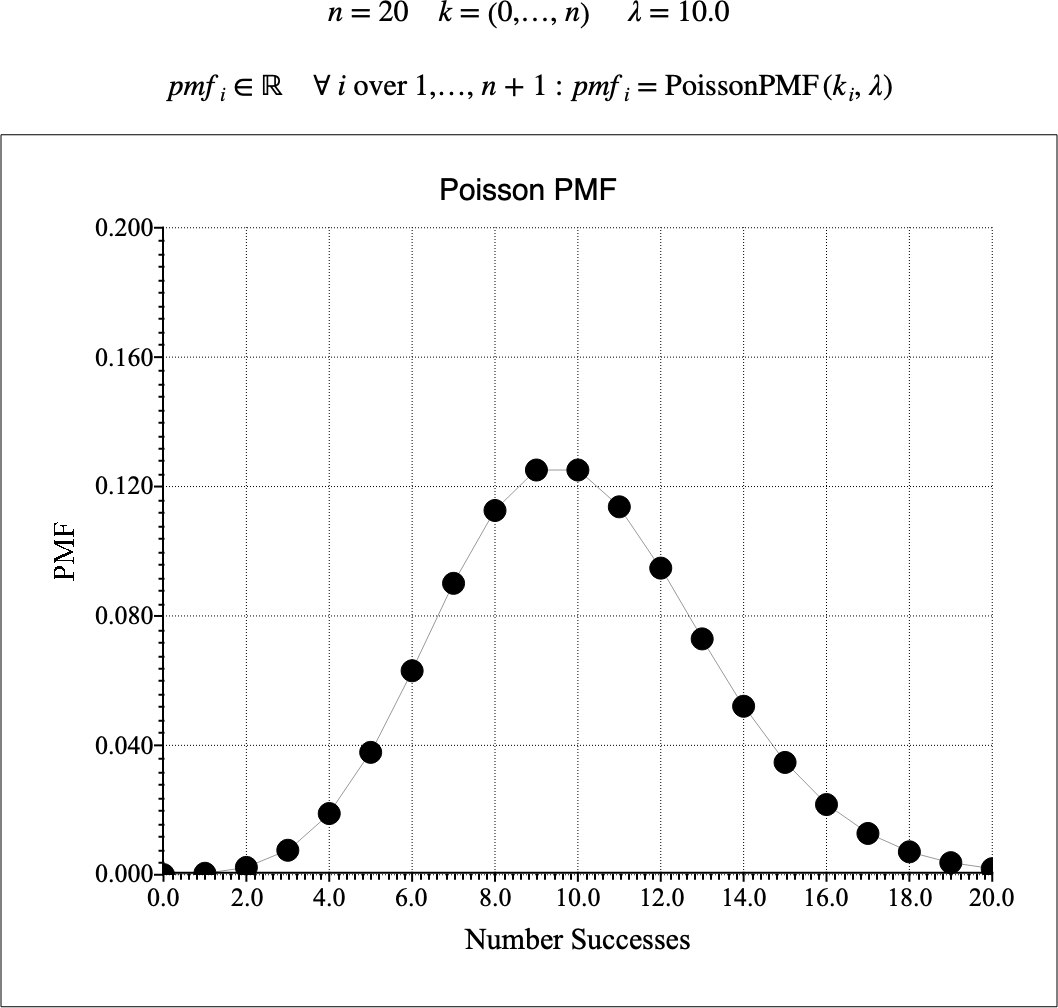# $$\text{PoissonPDF}$$¶

You can use the $$\text{PoissonPDF}$$ function to calculate the probability mass function (PMF) of the Poisson distribution.

You can use the \poissonp backslash command to insert this function.

The following variants of this function are available:

• $$\text{real } \text{PoissonPMF} \left ( \text{<k>}, \text{<}\lambda\text{>} \right )$$

Where $$k$$, and $$\lambda$$ are scalar values representing the value of interest (number of events), and the rate. Note that this function is defined over the range $$k \geq 0$$ and $$\lambda > 0$$. The $$\text{PoissonPMF}$$ function will generate a run-time error or return NaN for values for which the function is not defined.

The value is calculated directly using the relation:

$\text{PoissonPMF} \left ( k, \lambda \right ) = \frac{\lambda ^ k e ^ {-\lambda}}{k !}$

The Poisson distribution models the the number of events over a given time time period caused by a memoryless process and, in this respect, is closely related to the exponential distribution that models time between events assuming a memoryless process.

Figure 185 shows the basic use of the $$\text{PoissonPMF}$$ function.Figure 185 Example Use Of the PoissonPMF Function